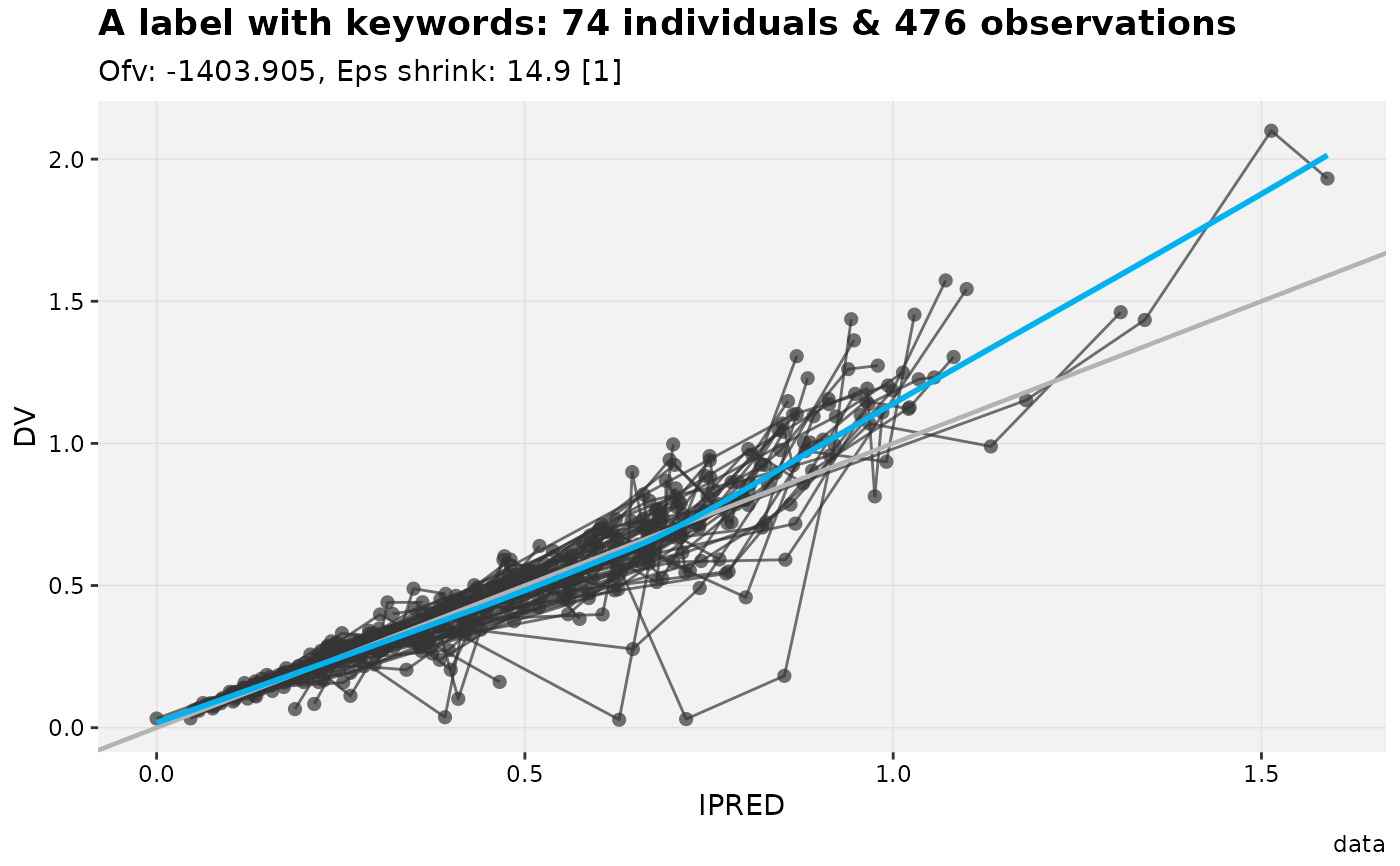This function explicitly draw an xpose_plot and interprets keywords contained in labels.

# S3 method for xpose_plot
print(x, page, ...)

## Arguments

x An xpose_plot object. The page number to be drawn. Can be specified as vector or range of integer values. Options to be passed on to the ggplot2 print method.

## Examples

my_plot <- dv_vs_ipred(xpdb_ex_pk) +
labs(title = 'A label with keywords: @nind individuals & @nobs observations')#> Using data from \$prob no.1#> Filtering data by EVID == 0# Using the print function
print(my_plot)#> geom_smooth() using formula 'y ~ x'#> geom_smooth() using formula 'y ~ x'# Or simply by writting the plot object name
my_plot#> geom_smooth() using formula 'y ~ x'#> geom_smooth() using formula 'y ~ x'